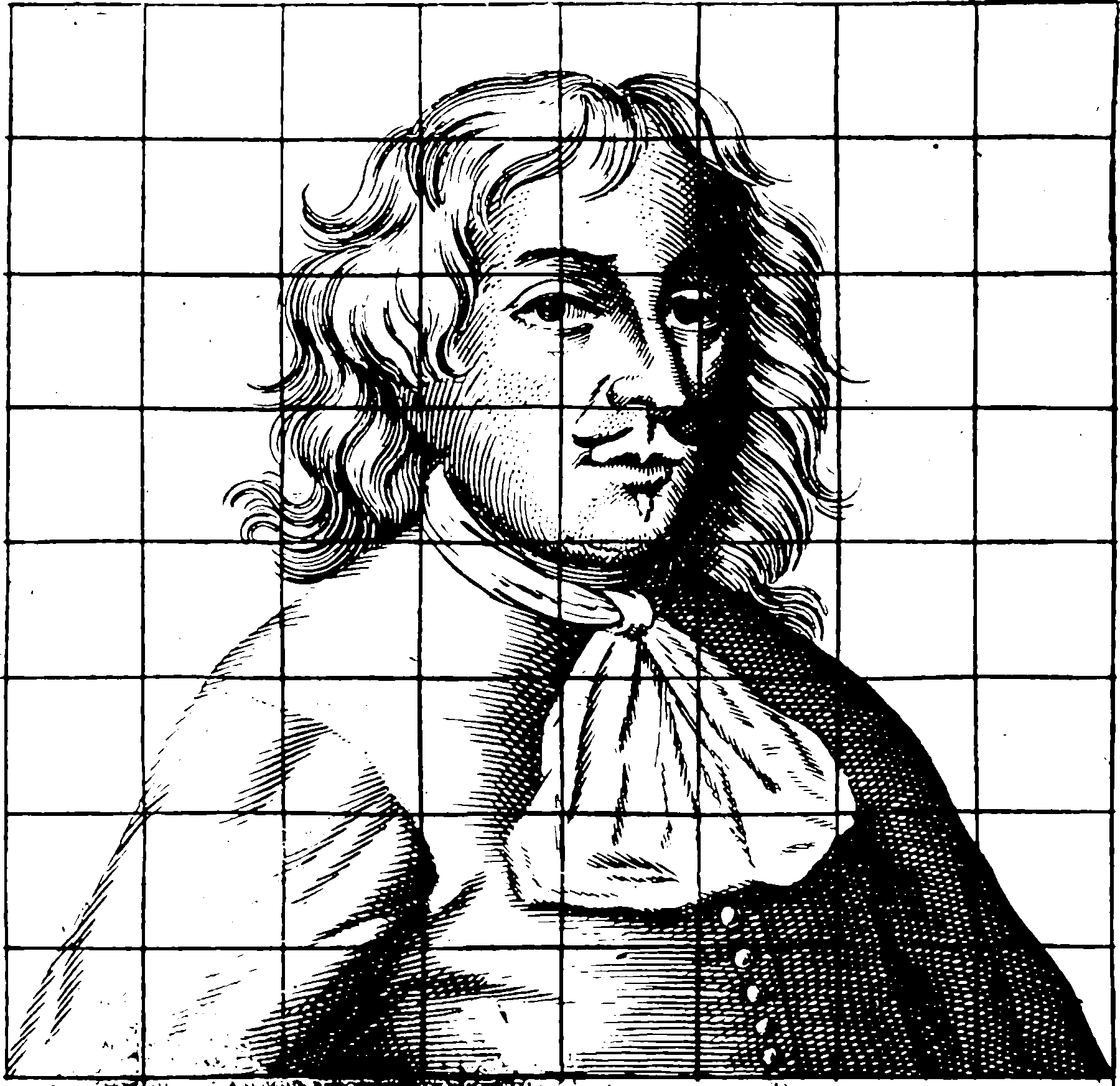# Signal sampling

## Discrete representation of continuous signals and converseWhen we can approximate discrete systems with continuous ones and vice versa. Sampling theorems. Nyquist rates, Compressive sampling, nonuniform signal sampling, signatures of rough paths, etc.

TODO: just write this as a basis decomp.

There are a few ways to frame this. Traditionally in electrical engineering applications we talk about Shannon sampling theorems, Nyquist rates and so on. The received-wisdom version of the Shannon theorem is that you can reconstruct a signal if you know it has frequencies in it that are “too high”. Specifically, if you sample a continuous time signal at intervals of $$T$$ seconds, then you had better have no frequencies of period shorter than $$2T$$.1 If you do much non-trivial signal processing, (in my case I constantly need to do things like multiplying signals) it rapidly becomes impossible to maintain bounds on the support of the spectrogram (TODO explain this with diagrams).

This doesn’t tell us much about more bizarre non-uniform sampling regimes, mild violations of frequency constraints, or whether other sets of (perhaps more domain-appropriate) constraints on our signals will lead to a sensible reconstruction theory.

More abstractly there is a Hilbert-space framing of this problem This way is general, and based on projections between Hilbert spaces. Nice works in this tradition are, e.g. that observes that you don’t care about Fourier spectrogram support, but rather the rate of degrees of freedom to construct a coherent sampling theory. Also accessible is , which constructs the problem of discretising signals as a minimal-loss projection/reconstruction problem.

More recently you have fancy persons such as Adcock and Hansen unifying compressed sensing and signal sampling with more or less the same framework. Looks interesting.

Most of the above use some variant of minimum $$L_2$$ norm error when reconstructing the signal. However, there are more reconstruction errors; for example I might wish to find some representation of a signal which is best with respect to some kind of transformation, e.g. in an inverse problem.

Or I might wish to sample a random signal, which is especially useful in functional Bayes inverse problems.1. I’m playing fast-and-loose with definitions here — the spectrum in this context is the continuous Fourier spectrogram.↩︎

### No comments yet. Why not leave one?

GitHub-flavored Markdown & a sane subset of HTML is supported.